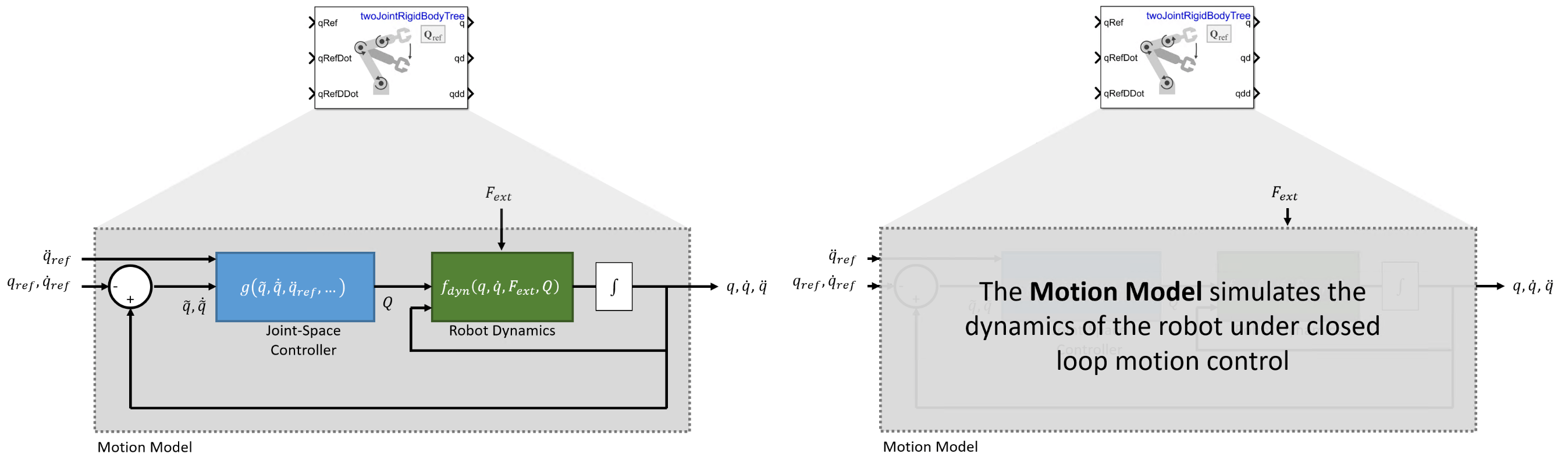Joint-Space Motion Model

The joint-space motion model characterizes the closed-loop motion of a manipulator under joint-space control, where the control action is defined in the joint configuration space with respect to the desired joint configuration. This topic covers the variables and equations for calculating joint-space motion control used in the jointSpaceMotionModel object. For task-space motion models, see the Plan and Execute Task- and Joint-Space Trajectories Using KINOVA Gen3 Manipulator object.

Types of joint-space control:For an example that covers the difference between task-space and joint-space control, see Plan and Execute Task- and Joint-Space Trajectories Using KINOVA Gen3 Manipulator.

Th joint-space motion model has the following state:

• $q$ — Robot joint configuration vector containing the array of joint positions defined in $\mathrm{rad}$ for revolute and $\mathit{m}$ for prismatic joints

• $\underset{}{\overset{˙}{q}}$ — Vector of joint velocities in $\frac{\mathrm{rad}}{\mathit{s}}$ and $\frac{\mathit{m}}{\mathit{s}}$

• $\underset{}{\overset{¨}{q}}$ — Vector of joint accelerations in $\frac{\mathrm{rad}}{{\mathit{s}}^{2}}$ or $\frac{\mathit{m}}{{\mathit{s}}^{2}}$

The motion model includes three ways to model and control the overall behavior:

• Independent Joint Motion: Each joint is modeled independently as a second-order system. This model may be considered a best-case scenario for how closed-loop motion may behave since the dynamics are simplified and directly prescribed.

• Computed Torque Control: The rigid body dynamics are modeled using the standard equations of motion, but compensating for the full-body dynamics and assigning error dynamics. This is a higher-fidelity version of the independent joint motion control.

• PD Control: The rigid body dynamics are modeled using the standard equations of motion with a joint torque input given by proportional-derivative (PD) control. This model represents a controller that does not compensate tightly for the overall effects of rigid body motion.

To set these different control types, see the MotionType property on the jointSpaceMotionModel object

Equations of Motion

Independent Joint Motion

When performing inpdependent joint motion control, instead of modeling the robot with the standard rigid body dynamics, each joint is modeled as a second-order system. Therefore, the $\mathit{i}$-th joint has the following dynamics:

${\underset{}{\overset{¨}{\underset{}{\overset{\sim }{q}}}}}_{i}=-{\omega }_{n}^{2}{\underset{}{\overset{\sim }{q}}}_{i}-2\zeta {\omega }_{n}{\underset{}{\overset{˙}{\underset{}{\overset{\sim }{q}}}}}_{i}$

${\underset{}{\overset{\sim }{q}}}_{i}={q}_{i}-{q}_{i,ref}$

These parameters characterize the desired response defined for each joint:

• ${\omega }_{n}$ — the natural frequency specified in units of 1/s

• $\zeta$ — t the damping ratio, which is unitless

The manipulator dynamics are then modeled as:

$\frac{d}{dt}\left[\begin{array}{c}q\\ \underset{}{\overset{˙}{q}}\end{array}\right]=\left[\begin{array}{cc}0& I\\ {\left[-{\omega }_{n}^{2}\right]}_{diag}& {\left[-2\zeta {\omega }_{n}\right]}_{diag}\end{array}\right]\left[\begin{array}{c}q\\ \underset{}{\overset{˙}{q}}\end{array}\right]+\left[\begin{array}{cc}0& I\\ {\left[{\omega }_{n}^{2}\right]}_{diag}& {\left[2\zeta {\omega }_{n}\right]}_{diag}\end{array}\right]\left[\begin{array}{c}{q}_{ref}\\ {\underset{}{\overset{˙}{q}}}_{ref}\end{array}\right]$

The control input relies on these user-defined parameters:

• ${\left[-{\omega }_{n}^{2}\right]}_{diag}$ — Diagonal matrix where the $\left(\mathit{i},\mathit{i}\right)$-th element corresponds to the $\mathit{i}$-th element of the N-element vector of natural frequencies (NaturalFrequency) in Hz (${\mathit{s}}^{-1}\right)$

• ${\left[-2\zeta {\omega }_{n}^{2}\right]}_{diag}$ — Diagonal matrix where the $\left(\mathit{i},\mathit{i}\right)$-th element corresponds to a product of the natural frequencies and the damping ratios (DampingRatio) of the $\mathit{i}$-th element.

This model accepts the following inputs:

• ${q}_{ref}$ — Reference joint configuration vector containing the array of reference robot joint positions specified in $\mathrm{rad}$ for revolute and $\mathit{m}$ for prismatic joints

• ${\underset{}{\overset{˙}{q}}}_{ref}$ — Reference joint velocities vector, in rad/s or m/s for revolute and prismatic joints, respectively

The values of ${\omega }_{n}$ iand $\zeta$ may be set directly, or they may be provided using the updateErrorDynamicsFromStep object function, which computes values for ${\omega }_{n}$ iand $\zeta$ based on desired unit step response.

Computed Torque Control

With computed torque control, the motion model uses standard rigid body dynamics, but with the torque input $\tau$ given by a control law that compensates for the rigid body dynamics and instead assigns a second-order dynamics response:

$\frac{d}{dt}\left[\begin{array}{c}q\\ \underset{}{\overset{˙}{q}}\end{array}\right]={f}_{dyn}\left(q,\underset{}{\overset{˙}{q}},\tau ,{F}_{ext}\right)$

$\tau =M\left(q\right){a}_{a}+C\left(q,\underset{}{\overset{˙}{q}}\right)\underset{}{\overset{˙}{q}}+G\left(q\right)$

${a}_{q}={\underset{}{\overset{¨}{q}}}_{ref}-{\left[{\omega }_{n}^{2}\right]}_{diag}\underset{}{\overset{\sim }{q}}-{\left[2\zeta {\omega }_{n}\right]}_{diag}\underset{}{\overset{˙}{\underset{}{\overset{\sim }{q}}}}$

${\underset{}{\overset{\sim }{q}}}_{i}=q-{q}_{ref}$

where,

• $M\left(q\right)$ — Joint-space mass matrix based on the current robot configuration calculated by the massMatrix object function.

• $C\left(q,\underset{}{\overset{˙}{q}}\right)$ — Coriolis terms, which when multiplied by $\underset{}{\overset{˙}{q}}$ results in the velocity product calculated by the velocityProduct object function.

• $G\left(q\right)$ — Gravity torques and forces for all joints to maintain their positions in the specified gravity (Gravity) calculated by the gravityTorque object function.

The control input relies on these user-defined parameters:

• ${\left[-{\omega }_{n}^{2}\right]}_{diag}$ — Diagonal matrix where the $\left(\mathit{i},\mathit{i}\right)$-th element corresponds to the $\mathit{i}$-th element of the N-element vector of natural frequencies (NaturalFrequency) in Hz (${\mathit{s}}^{-1}\right)$

• ${\left[-2\zeta {\omega }_{n}^{2}\right]}_{diag}$ — Diagonal matrix where the $\left(\mathit{i},\mathit{i}\right)$-th element corresponds to a product of the natural frequencies and the damping ratios (DampingRatio) of the $\mathit{i}$-th element.

This model accepts ${q}_{ref},{\underset{}{\overset{˙}{q}}}_{ref},{\underset{}{\overset{¨}{q}}}_{ref}$ as the desired reference joint configuration, velocities, and accelrations.

Because the dynamics are compensated, no external forces should be felt. The computed torque control motion model behaves the same as the independent joint motion model.

As with independent joint motion, the values of ${\omega }_{n}$ iand $\zeta$ may be set directly, or they may be provided using the the updateErrorDynamicsFromStep object function, which computes values for ${\omega }_{n}$ iand $\zeta$ based on desired unit step response.

Proportional-Derivative (PD) Control

With PD control, the robot models behavior according to standard rigid body dynamics, but with the torque input $\tau$ given by a control law that applies PD control based on the joint error and gravity compensation:

$\frac{d}{dt}\left[\begin{array}{c}q\\ \underset{}{\overset{˙}{q}}\end{array}\right]={f}_{dyn}\left(q,\underset{}{\overset{˙}{q}},\tau ,{F}_{ext}\right)$

$\tau =-{K}_{P}\left(q-{q}_{ref}\right)-{K}_{D}\left(\underset{}{\overset{˙}{q}}-{\underset{}{\overset{˙}{q}}}_{ref}\right)+G\left(q\right)$

where

• $G\left(q\right)$ — Gravity torques and forces for all joints to maintain their positions in the specified gravity (Gravity) calculated by the gravityTorque object function.

The control input relies on these user-defined parameters:

• ${K}_{P}$: Proportional gain, specified as an N-by-N matrix

• ${K}_{D}$: Derivative gain, specified as an N-by-N matrix

This model accepts ${q}_{ref},{\underset{}{\overset{˙}{q}}}_{ref}$ as the desired reference joint configuration and velocities.